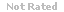Loading... Please wait...

A formal statement that there is an absence of relationship between variables when tested by a researcher is called:

Price:
\$24.99

Product Description

1. A formal statement that there is an absence of relationship between variables when tested by a researcher is called:
2. Bivariate statistics refers to the statistical analysis of the relationship between two variables.
3. Positive relationships between two variables indicate that, as the score of one increases, the score of the other increases.
4. A result that is probably not attributable to chance is:
5. A score that is likely to fall into the middle 68% of scores of a normal distribution will fall inside these values:
6. It is important to assess the magnitude or strength of a relationship because this assists you with deciding whether or not a variable A causes variable B.
7. In a negative relationship, as the score of one variable decreases, the score on the second variable decreases.
8. A set of subjects, usually randomly sampled, selected to participate in a research study is called:
9. A perfect negative relationship between two variables is expressed as r=0
10. When examining the relationship between a nominal variable and an interval or ratio variable, you would create a table using the nominal variables, calculate the mode and median of the interval or ratio variable, then make a decision regarding the relationship using the mode and median.
11. A Z score of +/-1.96 is equivalent to these values on a normal distribution.
12. An assumption used by statisticians which states that population parameters are normally distributed:
13. When examining the relationship between two interval or ration level variables, you can use a scatterplot to allow you to visualize the relationship between the two.
14. Correlation coefficients, r, range from -1.00 to +1.00.
15. An entire set of individuals or objects having some common characteristics is:
16. Data which uses number values, has an equal distance between values, and has a possible absolute zero is:
17. To determine the proportion of variability in one variable that can be accounted for or explained by the variability of a second variable, you would calculate a correlation coefficient, then square it.
18. A significant finding with the 2 sample t-test indicates a strong relationship exists between the 2 population means.
19. Data which uses number values with an equal distance between values are:
20. Data which uses numbers to provide categories or labels as well as the ordering of the categories are:
21. Not all variables retained in a regression model are required to be significant
22. Parametric tests can be used with any type of data.
23. This term refers to how data spreads out or disperses within a distribution.
24. Relevant data that are expressed in numerical form are called:
25. It is not necessary to look at the frequency distribution if the mean, median, and mode are known.
26. Causation is synonymous with association.
27. The area of the theoretical distribution where the researcher will reject the null hypothesis is called:
28. The arithmetic average of the data is called the:
29. Regression uses the least squares method to fit a model.
30. The difference between the highest and lowest score in a distribution is called:
31. This is the measurement of dispersion equal to the square root of the mathematical variance
32. This is used to compare means while controlling for the effect of a covariate.
33. The assumptions for ANCOVA differ substantially from those for ANOVA.
34. When the researcher incorrectly rejects the null hypothesis, it is known as:
35. You are studying the effect of hypnosis on weight loss. You will measure each patient's weight pre- and post-intervention. The most appropriate test is:
36. What is the minimum level of measurement appropriate for the independent variable in a t-test?
37. ANOVA assumes which of the following?
38. Public Health nurses want to compare the mean weight of US 6th graders to a sample of 6th graders in one school. Which statistical test is most appropriate
39. A group of neonatal nurses wanted to know if there was a difference in mean heart rate between premature babies who were swaddled and babies who were not swaddled. Babies were randomly assigned to swaddling or no swaddling. Which test is most appropriate?
40. Psych nurses wanted to evaluate the effect of three different treatments for anxiety - positive imagery, therapeutic touch, and medication on anxiety score (interval data) while controlling for the effect of length of stay. Which test is most appropriate?
41. Critical care nurses wanted to evaluate the effect of heparin vs saline IV flush solution on IV patency (patent vs not patent). Which test is most appropriate?
42. A nurse researcher wanted to evaluate blood pressure in a set of patients/research subjects at 1, 3, and 6 months. What test would be most appropriate?
43. In a study that examines the effect of a peer mentor on the heart rate of patients, what is the dependent variable?
44. A variable with equal distances between score units which also has a true meaningful zero is:
45. The standard deviation is:
46. If a researcher accepts a null hypothesis when that hypothesis is actually true, she has committed:
47. Chi-square tests can be conducted for a 4X3 contingency table
48. This is used when a conservative approach is desired and an expected cell frequency is less than 5 in a 2X2 table.
49. The distribution of chi-square values is NOT normally distributed
50. Generally, an ANOVA test is used when 3 or more variables are compared.

Customers Who Viewed This Product Also Viewed

• \$8.99
• \$20.00
• \$14.99
• \$12.99
• \$19.99

• \$24.99